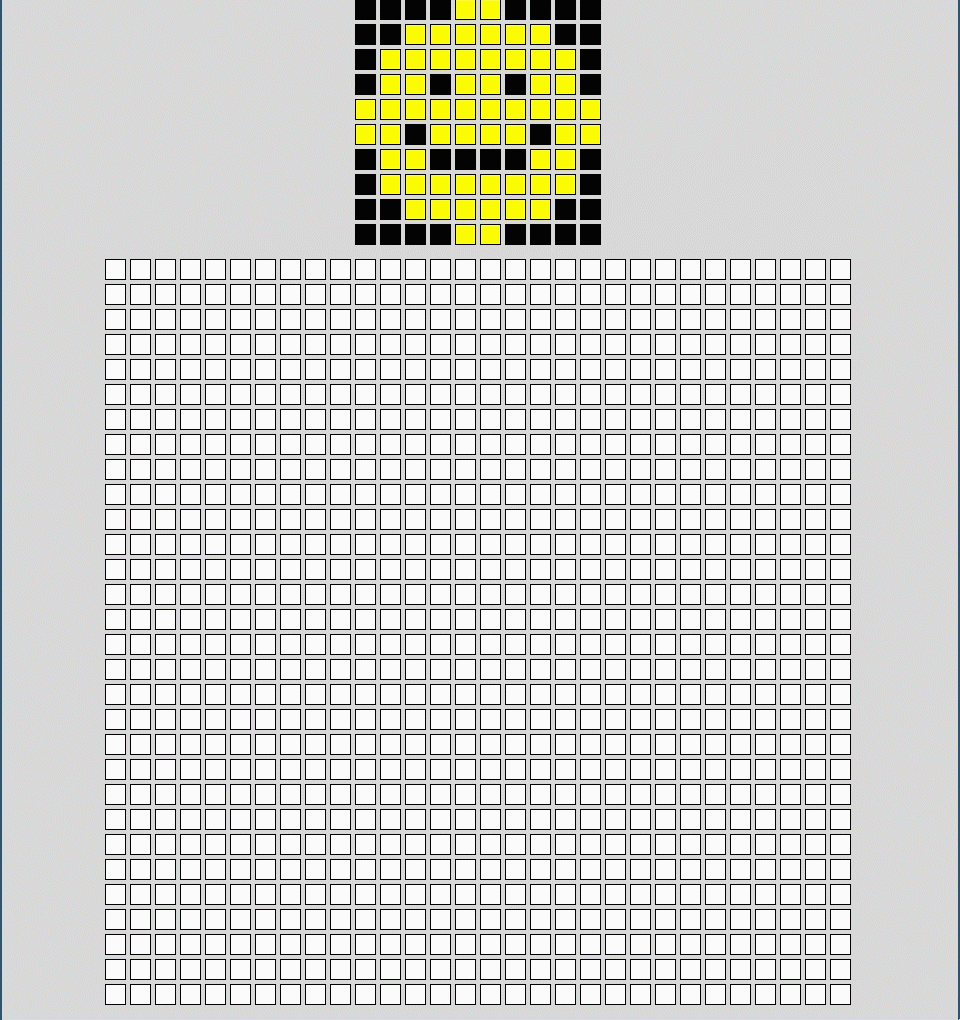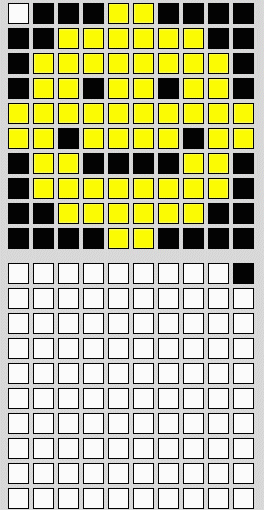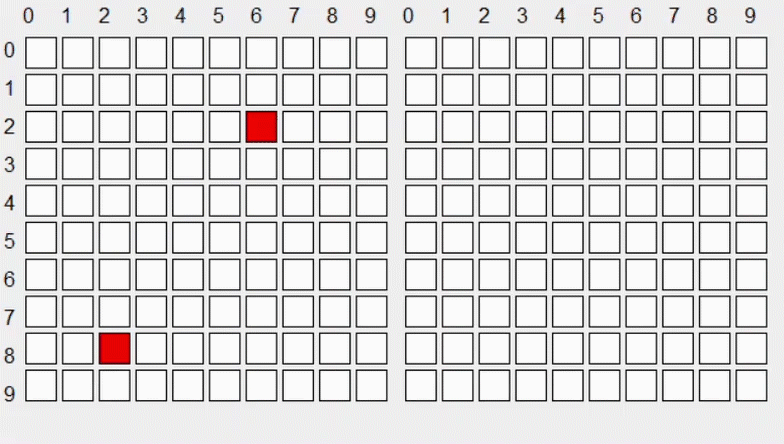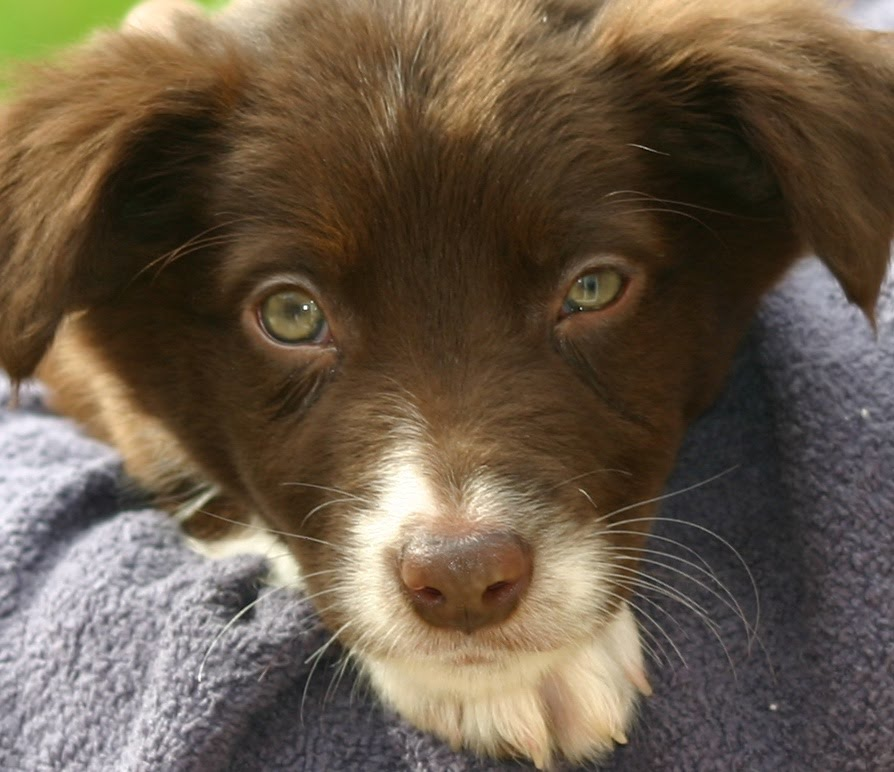## Want to keep learning?

This content is taken from the Raspberry Pi Foundation & National Centre for Computing Education's online course, Representing Data with Images and Sound: Bringing Data to Life. Join the course to learn more.
2.7

# Modifying images

Now that you know bitmap images are made up of tiny coloured pixels, in this and following steps you will dive into modifying these pixels and therefore, the pictures themselves — culminating in Instagram-style filters and blurry images galore! In this step, we’ll look at how to scale and rotate images, and I’ll explain a bit about each, before you get to create a Python script to rotate a bitmap image by moving the individual pixels.

## Scale

Scaling is simply resizing an image to make it bigger or smaller.When you scale up an image, the image grows and more pixels are required. There are many methods for creating a new, scaled-up image, and one of the simplest is ‘pixel replication’. As the name suggests, this method replicates pixels to scale up an image. We’ll now look at how it works with the help of the emoji you made earlier in the week.

bbbbyybbbb
bbyyyyyybb
byyyyyyyyb
byybyybyyb
yyyyyyyyyy
yybyyyybyy
byybbbbyyb
byyyyyyyyb
bbyyyyyybb
bbbbyybbbb


The pixels in the image can be copied so that the image becomes twice as wide and twice as tall: for every pixel, three copies are made and arranged with the ‘original’ pixel in a 2×2 grid. So the original first line of the emoji:

bbbbyybbbb


becomes two rows, each twice as long.

bbbbbbbbyyyybbbbbbbb
bbbbbbbbyyyybbbbbbbb


You can perform this kind of scaling in Python, re-using the code you wrote for displaying your emoji. The edited code is below, and it’s also available in this repl.it.

The only changes necessary are:

1. Setting a scale variable to decide the size of the new image
2. Making the new blank image the size of the enlarged image
3. Selecting the positions of new pixels in the enlarged image
from PIL import Image

b = (0,0,0)
y = (255,255,0)

face = [[b,b,b,b,y,y,b,b,b,b],
[b,b,y,y,y,y,y,y,b,b],
[b,y,y,y,y,y,y,y,y,b],
[b,y,y,b,y,y,b,y,y,b],
[y,y,y,y,y,y,y,y,y,y],
[y,y,b,y,y,y,y,b,y,y],
[b,y,y,b,b,b,b,y,y,b],
[b,y,y,y,y,y,y,y,y,b],
[b,b,y,y,y,y,y,y,b,b],
[b,b,b,b,y,y,b,b,b,b]]

scale = 50

scaled = Image.new("RGB", (scale * 10, scale * 10))

width, height = scaled.size

for row in range(height):
for col in range(width):
scaled.putpixel((col,row), face[int(row/scale)][int(col/scale)])

scaled.save('expanded_smiley.jpg')
scaled.show()

• Try out various images and scale values to solidify your understanding of how the code works.

How could you scale an image down? What problems might you encounter when trying to scale down a large complex image?

## Rotate

To rotate an image 90 degrees clockwise using Python, you need to look at each pixel of an image and copy its properties to a new destination pixel. Here the emoji from earlier is being rotated 90 degrees clockwise:To understand how to calulate the position of the pixel in the rotated image, have a look at this example:The red pixel begins at x = 6, y = 2. In the rotated image, it ends up at x = 7, y= 6. Another pixel, which begins at x = 2, y = 8, ends up at x = 1, y = 2.

You can probably see that when the image is being rotated, the original x coordinate of any given pixel becomes its new y coordinate.

A pixel’s new x coordinate is a little harder to figure out: you first need to take the width of the original image and subtract 1. Then subtract the pixel’s original y coordinate. The resulting value is the pixel’s new x coordinate.

So the algorithm for moving pixels to rotate an image is:

• rotated y = original x
• rotated x = rotated_image_width - 1 - original y

When we apply this algorithm to the pixels we looked at in the example above, we get the following results for a 10-pixel–wide rotated image:

original x original y rotated x rotated y
6 2 7 6
2 8 1 2

## Activity: create a script for rotating images

You’ll put this into practice using this adorable picture of a puppy. Right-click on the image below and save it to your code folder, or use this repl.it online starter project.### Setting up

In a new Python file (or in the repl.it starter project), begin by importing the PIL module again, and then load the original puppy image.

from PIL import Image

original = Image.open("puppy.bmp")


### Widths and heights

As you’ve done for your emoji before, find the puppy.bmp image’s width and height using the .size property of PIL’s Image object.

Then set the the size of the rotated image: the width of the original image will be the height of the rotated image, and the height of the original will become the width of the rotated image.

ori_width, ori_height = original.size
rot_height, rot_width = ori_width, ori_height


### Create a new blank image

Now that you know the size of the rotated image, create a blank image to build upon.

rotated = Image.new("RGB", (rot_width, rot_height))


### Place the pixels

Loop over the x and y coordinates of all the pixels in the original image to find the pixels’ colour.

for y in range(ori_height):
for x in range(ori_width):
pixel = original.getpixel((x, y))


Each pixel can then be placed the new image. As we discussed above, a pixel’s new x coordinate is the original width minus 1 minus its original y position.

for y in range(ori_height):
for x in range(ori_width):
pixel = original.getpixel((x, y))
rotated.putpixel((rot_width - 1 - y, x), pixel)


### View the image

Finish by saving your code and viewing the new image file.

rotated.save('rotated_puppy.jpg', 'JPEG')
rotated.show() ## DO NOT INCLUDE IN repl.it


Run your code and check that the puppy picture has been rotated.

## Challenges

Try to complete some of these tasks:

• Change the code within the loop to rotate the image anti-clockwise 90 degrees
• Rotate the image by 180 degrees
• Can you enlarge the image before rotating it?